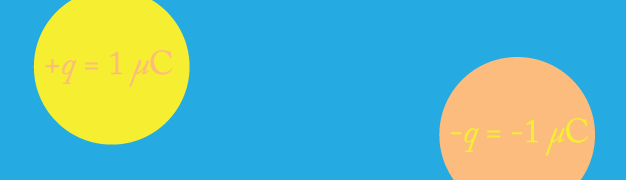# Opposite charges attractTwo point charges $+q=1~\mu\mbox{C}$ and $-q=-1~\mu\mbox{C}$ with mass $m=1~\mbox{g}$ are fixed at the positions $\pm \vec{r}_{0}$ with $|r_{0}|=1~\mbox{m}$. The charges are released from rest at $t=0$. Find the time $\tau$ in seconds at which they collide.

Hint: Can you do it without integrating by using Kepler's laws?

Details and assumptions

$k=\frac{1}{4\pi \epsilon_{0}}= 9\times 10^{9}~\mbox{m/F}$

×